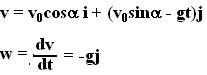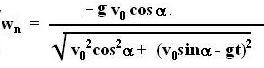## Wednesday, July 18, 2007

### Irodov Problem 1.30

We know that,The angle between v and w is given as,Having found the tangential component, now we can find the normal component knowing the magnitude of the acceleration is g.In the above equation we choose the -ive sign since we already know that g is acting in the -ive direction and cannot have a projection along the normal to the velocity. Thus,The velocity vector is directed along the tangent to the path of the particle, thus,These curves can now be plotted